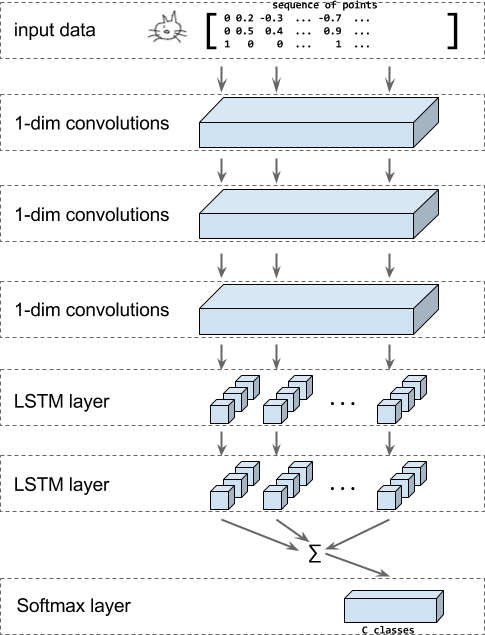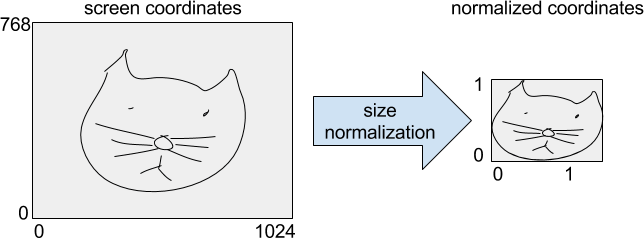# W3cubDocs

/TensorFlow Guide

# Recurrent Neural Networks for Drawing Classification

Quick, Draw! is a game where a player is challenged to draw a number of objects and see if a computer can recognize the drawing.

The recognition in Quick, Draw! is performed by a classifier that takes the user input, given as a sequence of strokes of points in x and y, and recognizes the object category that the user tried to draw.

In this tutorial we'll show how to build an RNN-based recognizer for this problem. The model will use a combination of convolutional layers, LSTM layers, and a softmax output layer to classify the drawings:The figure above shows the structure of the model that we will build in this tutorial. The input is a drawing that is encoded as a sequence of strokes of points in x, y, and n, where n indicates whether a the point is the first point in a new stroke.

Then, a series of 1-dimensional convolutions is applied. Then LSTM layers are applied and the sum of the outputs of all LSTM steps is fed into a softmax layer to make a classification decision among the classes of drawings that we know.

This tutorial uses the data from actual Quick, Draw! games that is publicly available. This dataset contains of 50M drawings in 345 categories.

## Run the tutorial code

To try the code for this tutorial:

1. Install TensorFlow if you haven't already.
3. Download the data in `TFRecord` format from here and unzip it. More details about how to obtain the original Quick, Draw! data and how to convert that to `TFRecord` files is available below.

4. Execute the tutorial code with the following command to train the RNN-based model described in this tutorial. Make sure to adjust the paths to point to the unzipped data from the download in step 3.

```python train_model.py \
--training_data=rnn_tutorial_data/training.tfrecord-?????-of-????? \
--eval_data=rnn_tutorial_data/eval.tfrecord-?????-of-????? \
--classes_file=rnn_tutorial_data/training.tfrecord.classes
```

## Tutorial details

We make the data that we use in this tutorial available as `TFRecord` files containing `TFExamples`. You can download the data from here:

Alternatively you can download the original data in `ndjson` format from the Google cloud and convert it to the `TFRecord` files containing `TFExamples` yourself as described in the next section.

The full Quick, Draw! dataset is available on Google Cloud Storage as ndjson files separated by category. You can browse the list of files in Cloud Console.

Then use the following command to check that your gsutil installation works and that you can access the data bucket:

```gsutil ls -r "gs://quickdraw_dataset/full/simplified/*"
```

which will output a long list of files like the following:

```gs://quickdraw_dataset/full/simplified/The Eiffel Tower.ndjson
gs://quickdraw_dataset/full/simplified/The Great Wall of China.ndjson
gs://quickdraw_dataset/full/simplified/The Mona Lisa.ndjson
gs://quickdraw_dataset/full/simplified/aircraft carrier.ndjson
...
```

```mkdir rnn_tutorial_data
cd rnn_tutorial_data
gsutil -m cp "gs://quickdraw_dataset/full/simplified/*" .
```

### Optional: Converting the data

To convert the `ndjson` files to TFRecord files containing `tf.train.Example` protos run the following command.

```python create_dataset.py --ndjson_path rnn_tutorial_data \
--output_path rnn_tutorial_data
```

This will store the data in 10 shards of TFRecord files with 10000 items per class for the training data and 1000 items per class as eval data.

This conversion process is described in more detail in the following.

The original QuickDraw data is formatted as `ndjson` files where each line contains a JSON object like the following:

```{"word":"cat",
"countrycode":"VE",
"timestamp":"2017-03-02 23:25:10.07453 UTC",
"recognized":true,
"key_id":"5201136883597312",
"drawing":[
[
[130,113,99,109,76,64,55,48,48,51,59,86,133,154,170,203,214,217,215,208,186,176,162,157,132],
[72,40,27,79,82,88,100,120,134,152,165,184,189,186,179,152,131,114,100,89,76,0,31,65,70]
],[
[76,28,7],
[136,128,128]
],[
[76,23,0],
[160,164,175]
],[
[87,52,37],
[175,191,204]
],[
[174,220,246,251],
[134,132,136,139]
],[
[175,255],
[147,168]
],[
[171,208,215],
[164,198,210]
],[
[130,110,108,111,130,139,139,119],
[129,134,137,144,148,144,136,130]
],[
[107,106],
[96,113]
]
]
}
```

For our purpose of building a classifier we only care about the fields "`word`" and "`drawing`". While parsing the ndjson files, we process them line by line using a function that converts the strokes from the `drawing` field into a tensor of size `[number of points, 3]` containing the differences of consecutive points. This function also returns the class name as a string.

```def parse_line(ndjson_line):
"""Parse an ndjson line and return ink (as np array) and classname."""
class_name = sample["word"]
inkarray = sample["drawing"]
stroke_lengths = [len(stroke) for stroke in inkarray]
total_points = sum(stroke_lengths)
np_ink = np.zeros((total_points, 3), dtype=np.float32)
current_t = 0
for stroke in inkarray:
for i in [0, 1]:
np_ink[current_t:(current_t + len(stroke)), i] = stroke[i]
current_t += len(stroke)
np_ink[current_t - 1, 2] = 1  # stroke_end
# Preprocessing.
# 1. Size normalization.
lower = np.min(np_ink[:, 0:2], axis=0)
upper = np.max(np_ink[:, 0:2], axis=0)
scale = upper - lower
scale[scale == 0] = 1
np_ink[:, 0:2] = (np_ink[:, 0:2] - lower) / scale
# 2. Compute deltas.
np_ink = np_ink[1:, 0:2] - np_ink[0:-1, 0:2]
return np_ink, class_name
```

Since we want the data to be shuffled for writing we read from each of the category files in random order and write to a random shard.

For the training data we read the first 10000 items for each class and for the eval data we read the next 1000 items for each class.

This data is then reformatted into a tensor of shape `[num_training_samples, max_length, 3]`. Then we determine the bounding box of the original drawing in screen coordinates and normalize the size such that the drawing has unit height.Finally, we compute the differences between consecutive points and store these as a `VarLenFeature` in a tensorflow.Example under the key `ink`. In addition we store the `class_index` as a single entry `FixedLengthFeature` and the `shape` of the `ink` as a `FixedLengthFeature` of length 2.

### Defining the model

To define the model we create a new `Estimator`. If you want to read more about estimators, we recommend this tutorial.

To build the model, we:

1. reshape the input back into the original shape - where the mini batch is padded to the maximal length of its contents. In addition to the ink data we also have the lengths for each example and the target class. This happens in the function `_get_input_tensors`.

2. pass the input through to a series of convolution layers in `_add_conv_layers`.

3. pass the output of the convolutions into a series of bidirectional LSTM layers in `_add_rnn_layers`. At the end of that, the outputs for each time step are summed up to have a compact, fixed length embedding of the input.

4. classify this embedding using a softmax layer in `_add_fc_layers`.

In code this looks like:

```inks, lengths, targets = _get_input_tensors(features, targets)
```

### _get_input_tensors

To obtain the input features we first obtain the shape from the features dict and then create a 1D tensor of size `[batch_size]` containing the lengths of the input sequences. The ink is stored as a SparseTensor in the features dict which we convert into a dense tensor and then reshape to be `[batch_size, ?, 3]`. And finally, if targets were passed in we make sure they are stored as a 1D tensor of size `[batch_size]`

In code this looks like this:

```shapes = features["shape"]
lengths = tf.squeeze(
tf.slice(shapes, begin=[0, 0], size=[params["batch_size"], 1]))
inks = tf.reshape(
tf.sparse_tensor_to_dense(features["ink"]),
[params["batch_size"], -1, 3])
if targets is not None:
targets = tf.squeeze(targets)
```

The desired number of convolution layers and the lengths of the filters is configured through the parameters `num_conv` and `conv_len` in the `params` dict.

The input is a sequence where each point has dimensionality 3. We are going to use 1D convolutions where we treat the 3 input features as channels. That means that the input is a `[batch_size, length, 3]` tensor and the output will be a `[batch_size, length, number_of_filters]` tensor.

```convolved = inks
for i in range(len(params.num_conv)):
convolved_input = convolved
if params.batch_norm:
convolved_input = tf.layers.batch_normalization(
convolved_input,
training=(mode == tf.estimator.ModeKeys.TRAIN))
# Add dropout layer if enabled and not first convolution layer.
if i > 0 and params.dropout:
convolved_input = tf.layers.dropout(
convolved_input,
rate=params.dropout,
training=(mode == tf.estimator.ModeKeys.TRAIN))
convolved = tf.layers.conv1d(
convolved_input,
filters=params.num_conv[i],
kernel_size=params.conv_len[i],
activation=None,
strides=1,
name="conv1d_%d" % i)
return convolved, lengths
```

We pass the output from the convolutions into bidirectional LSTM layers for which we use a helper function from contrib.

```outputs, _, _ = contrib_rnn.stack_bidirectional_dynamic_rnn(
cells_fw=[cell(params.num_nodes) for _ in range(params.num_layers)],
cells_bw=[cell(params.num_nodes) for _ in range(params.num_layers)],
inputs=convolved,
sequence_length=lengths,
dtype=tf.float32,
scope="rnn_classification")
```

see the code for more details and how to use `CUDA` accelerated implementations.

To create a compact, fixed-length embedding, we sum up the output of the LSTMs. We first zero out the regions of the batch where the sequences have no data.

```mask = tf.tile(
[1, 1, tf.shape(outputs)])
outputs = tf.reduce_sum(zero_outside, axis=1)
```

The embedding of the input is passed into a fully connected layer which we then use as a softmax layer.

```tf.layers.dense(final_state, params.num_classes)
```

### Loss, predictions, and optimizer

Finally, we need to add a loss, a training op, and predictions to create the `ModelFn`:

```cross_entropy = tf.reduce_mean(
tf.nn.sparse_softmax_cross_entropy_with_logits(
labels=targets, logits=logits))
train_op = tf.contrib.layers.optimize_loss(
loss=cross_entropy,
global_step=tf.train.get_global_step(),
learning_rate=params.learning_rate,
# some gradient clipping stabilizes training in the beginning.
predictions = tf.argmax(logits, axis=1)
return model_fn_lib.ModelFnOps(
mode=mode,
predictions={"logits": logits,
"predictions": predictions},
loss=cross_entropy,
train_op=train_op,
eval_metric_ops={"accuracy": tf.metrics.accuracy(targets, predictions)})
```

### Training and evaluating the model

To train and evaluate the model we can rely on the functionalities of the `Estimator` APIs and easily run training and evaluation with the `Experiment` APIs:

```estimator = tf.estimator.Estimator(
model_fn=model_fn,
model_dir=output_dir,
config=config,
params=model_params)
# Train the model.
tf.contrib.learn.Experiment(
estimator=estimator,
train_input_fn=get_input_fn(
mode=tf.contrib.learn.ModeKeys.TRAIN,
tfrecord_pattern=FLAGS.training_data,
batch_size=FLAGS.batch_size),
train_steps=FLAGS.steps,
eval_input_fn=get_input_fn(
mode=tf.contrib.learn.ModeKeys.EVAL,
tfrecord_pattern=FLAGS.eval_data,
batch_size=FLAGS.batch_size),
min_eval_frequency=1000)
```

Note that this tutorial is just a quick example on a relatively small dataset to get you familiar with the APIs of recurrent neural networks and estimators. Such models can be even more powerful if you try them on a large dataset.

When training the model for 1M steps you can expect to get an accuracy of approximately of approximately 70% on the top-1 candidate. Note that this accuracy is sufficient to build the quickdraw game because of the game dynamics the user will be able to adjust their drawing until it is ready. Also, the game does not use the top-1 candidate only but accepts a drawing as correct if the target category shows up with a score better than a fixed threshold.